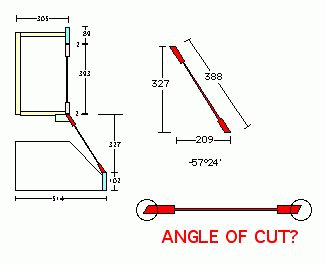# Figuring Angles: Degrees Versus Minutes

A little help with confusing geometry notation — how to convert "minutes" of angle to decimals. April 14, 2010

Question
I need to fabricate a hood fan cabinet that follows the math below. According to the CAD drawing, the angle of the front panel is 57ş24'. (I have no idea what the 24' part means. If anybody knows, please jump in.) I am trying to calculate the angle for horizontal cuts at top and bottom of the panel. Would this angle be 90 - 57.24? Is there a simple formula for inputting rise and run in order to calculate an angle?Forum Responses
(Cabinetmaking Forum)
From contributor M:
24' means 24 minutes. 1 minute = 1/60th of a degree, so 24 minutes = 24/60 = .40 degrees. I'm guessing 57-1/2 would be close enough.

Yes, the cut angle is 90 - 57.40 = 32.60.

The only formula is tangent 57.40 = rise/run.

Based on your questions, I'm guessing your trig skills aren't real up to date, so this probably doesn't help.

Specific to this project, the tangent of 57.40 = 1.564, so rise = run x 1.564.

I think the easiest way is just to lay it out in some type of drawing program.

From contributor J:
You have the angle measurement in your CAD program set to display DMS (degrees, minutes, seconds). You should change it to read a decimal, as it's a little easier to comprehend.

So your reading is 57 degrees and 24 minutes or 57 degrees and 24/60th of a degree.

From the original questioner:
Thanks for the explanation about the minutes, and the tip about angle settings in my CAD program. I will look into that.